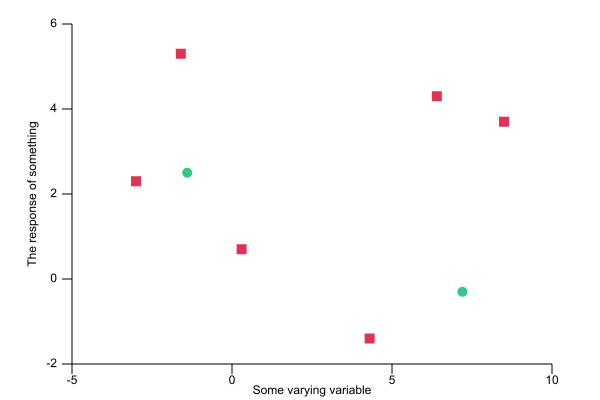## plotlib

Pure Rust plotting library

### 6 releases(breaking)

 0.5.1 Mar 28, 2020 Mar 14, 2020 Mar 2, 2019 Mar 1, 2018 Mar 9, 2017

#74 in Visualization

Used in fewer than 14 crates

105KB
2.5K SLoC

# plotlib`plotlib` is a generic data visualisation and plotting library for Rust. It is currently in the very early stages of development.

It can currently produce:

• histograms
• scatter plots
• line graphs from data or from function definitions
• box plots
• bar charts

rendering them as either SVG or plain text.

The API is still very much in flux and is subject to change.

For example, code like:

``````use plotlib::page::Page;
use plotlib::repr::Plot;
use plotlib::view::ContinuousView;
use plotlib::style::{PointMarker, PointStyle};

fn main() {
// Scatter plots expect a list of pairs
let data1 = vec![
(-3.0, 2.3),
(-1.6, 5.3),
(0.3, 0.7),
(4.3, -1.4),
(6.4, 4.3),
(8.5, 3.7),
];

// We create our scatter plot from the data
let s1: Plot = Plot::new(data1).point_style(
PointStyle::new()
.marker(PointMarker::Square) // setting the marker to be a square
.colour("#DD3355"),
); // and a custom colour

// We can plot multiple data sets in the same view
let data2 = vec![(-1.4, 2.5), (7.2, -0.3)];
let s2: Plot = Plot::new(data2).point_style(
PointStyle::new() // uses the default marker
.colour("#35C788"),
); // and a different colour

// The 'view' describes what set of data is drawn
let v = ContinuousView::new()
.x_range(-5., 10.)
.y_range(-2., 6.)
.x_label("Some varying variable")
.y_label("The response of something");

// A page with a single view is then saved to an SVG file
Page::single(&v).save("scatter.svg").unwrap();
}

``````

will produce output like:~135KB